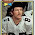## Sunday, September 25, 2016

### 49 61 63 102 | The death of Bill Nunn, (Radio Raheem from Do the Right Thing), September 24, 2016

William Goldwyn Nunn = 89
Do the Right Thing came out in '89

http://www.imdb.com/title/tt0097216/

William = 5+9+3+3+9+1+4 = 34 (Bill = 2+9+3+3 = 17)
Goldwyn = 7+6+3+4+5+7+5 = 37
Nunn = 5+3+5+5 = 18
III = 9+9+9 = 27

William Goldwyn Nunn III = 116
Bill Nunn = 35

William = 23+9+12+12+9+1+13 = 79 (Bill = 2+9+12+12 = 35)
Goldwyn = 7+15+12+4+23+25+14 = 100
Nunn =14+21+14+14 = 63 (When you sum 1-63, it totals 2016)
III = 9+9+9 = 27

William Goldwyn Nunn III = 269
Bill Nunn = 98

He is most remembered for the character Radio Raheem, in the film Do the Right Thing, which ends with the wrong thing happening.

The name Radio Raheem connects to '61', like the year the Civil War Began, April 12, 1861, the 102nd day of the year.  '102' connects to the current Charlotte Race Riots.

10/20/1953 = 10+20+19+53 = 102 (Charlotte) (September 20, day that leaves 102-days left in year)
10/20/1953 = 10+20+(1+9+5+3) = 48
10/20/1953 = 1+0+2+0+1+9+5+3 = 21
10/20/53 = 10+20+53 = 83 (Football)

The name Radio Raheem also connects to this years' 97th season of the NFL, 'football'.

9/24/2016 = 9+24+20+16 = 69
9/24/2016 = 9+24+(2+0+1+6) = 42 (Freemason) (February) (Nigger)
9/24/2016 = 9+2+4+2+0+1+6 = 24
9/24/16 = 9+24+16 = 49

'Bill' died 26-days, or 3-weeks and 5-days before his 63rd birthday.

Notice it says he was 63-years old.  He was not, he was 62, just short of his 63rd birthday.

Of course, when you sum 1-63, it totals 2016.

Notice Spike Lee is wearing 49ers gear for the death.  Again, he died on a date with '49' numerology.

9/24/16 = 9+24+16 = 49

1.(Of course, when you sum 1-63, it totals 2016)..... I'm just admitting I dont even know what that means or how it works

1.1 through 63.

1+2+3+4..all the way to 63.

2.oh,well,ok lol

3.Yea.. It's how you get the "triangular" number. I just found that out. So 2016 is the 63rd triangular number.

2.1.Np..that method of stack adding must be how i got 81 seasons for the Chargers. Lol

3.Fuuuuuuuuuuuuc Spike Lee, I can't stand that non-illuminated bastard

4.Fuuuuuuuuuuuuc Spike Lee, I can't stand that non-illuminated bastard

5.Thanks zac I immediately thought something was up that's why I asked about it I'm trying to learn the system also keep teaching I'm locked in

6.Thanks zac I immediately thought something was up that's why I asked about it I'm trying to learn the system also keep teaching I'm locked in